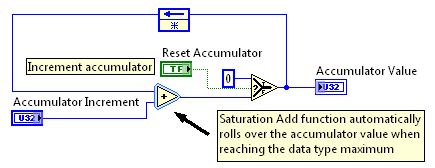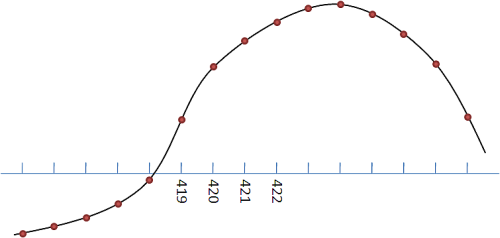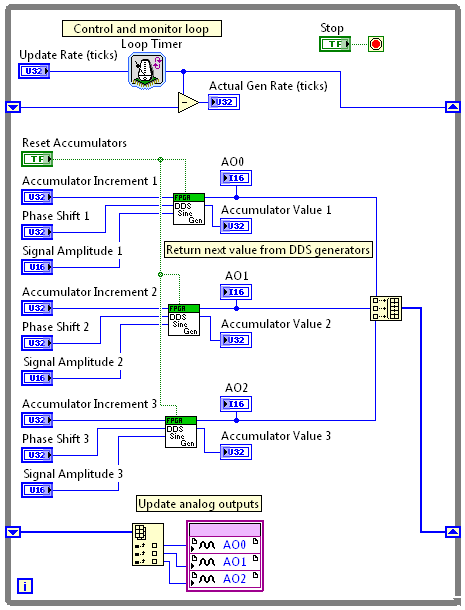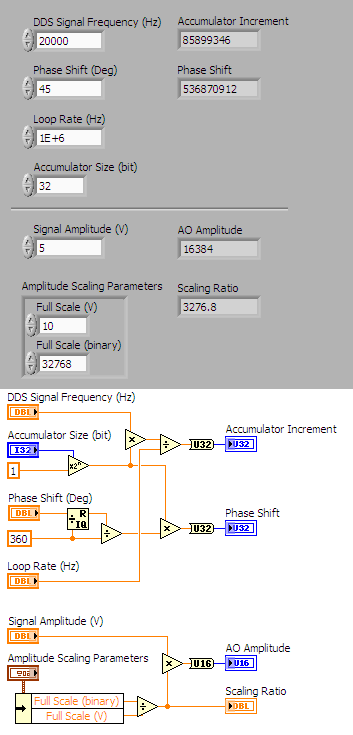# Example Code

## Overview

One common method used to generate repetitive, arbitrary waveforms with high degrees of frequency and phase control is direct digital synthesis (DDS). This reference design shows how you can easily add a DDS waveform generator to your LabVIEW FPGA application and output waveforms with milliHz or better relative frequency control as well as precise phase control between multiple waveforms.

This content has been archived and is no longer being monitored or maintained. Please use the code with caution.

The examples for this article are contained in a LabVIEW 2012 project in the ZIP file above. Unzip the file on your local hard drive and open the LabVIEW project file.

## Overview

The reference design and example presented in this article illustrates how you can add a DDS (direct digital synthesis) waveform generator to your LabVIEW FPGA-based application. By specifying your own reference waveform in a look-up table you can generate any waveform using the DDS framework.

These waveforms are specified point-by-point and can be any shape within the restrictions of the hardware that is generating the signal. It is possible to integrate glitches, drift, noise, and other anomalies in a signal that allows for the testing of real-world conditions that a device might encounter when not in a controlled environment..

## DDS Theory

The core component of a DDS waveform generator is the accumulator. The accumulator is a running counter which stores the current phase value of the generated waveform. The rate at which the accumulator is updated and the accumulator increment value determine the frequency of the generated waveform. For example if the accumulator is updated 360 times per second and the accumulator increment is one degree, then the generated frequency is 1 Hz (360 degrees per second). When the accumulator phase value reaches the maximum (360 degrees) it rolls over and starts again at 0 degrees. In order to represent the phase value more accurately the accumulator commonly uses 32-, 48-, or 64-bit for the counter. In a 32-bit accumulator the phase value has a range from 0 to 4294967295 which represents one full cycle of the reference waveform or 0 to 360 degrees.

The current accumulator (phase) value is used to perform a lookup operation in a lookup table of the reference waveform to determine the next output value. The lookup table contains one cycle of the waveform to be generated and typically contains 1024 to 8192 sample points which represent the waveform.

Because the accumulator value typically has a lot more resolution than the reference waveform, limited by the number of samples, the lookup operation may also perform an interpolation between two samples in the reference waveform. This is done based on the extra resolution in the accumulator value and returns a more accurate update value which provides better frequency control and less harmonic distortion in the generated signal.

A more detailed description of DDS can be found in Understanding Direct Digital Synthesis (DDS).

## FPGA DDS Implementation

The LabVIEW project included in this article contains several FPGA examples that use a sine and triangle DDS generator VI. These VIs can be reused without changes in your own application or can be adapted to the needs of your application.

The core of the FPGA DDS generator VIs (FPGA DDS SineGen IP.vi, FPGA DDS TriangleGen IP.vi) are the accumulator and waveform lookup.

### Accumulator

The accumulator in the DDS generator is a 32-bit counter. Each time the DDG generator VI is called the accumulator increases the current accumulator phase value by the specified Accumulator Increment. The Saturation Add function automatically wraps so that when the maximum phase value is reached the accumulator value rolls over back to 0. The DDS generator VI includes a Reset input which reinitializes the Accumulator Value. This can be used to synchronize multiple DDS generators used in the same application VI.Figure 1: DDS Accumulator implemented in LabVIEW FPGA

### Waveform Lookup

The waveform lookup portion of the DDS generator uses the current accumulator phase value to return the current waveform value from a reference waveform look-up table. The reference waveform is easily stored using the configurable LabVIEW FPGA Look-up Table function. You can define the size of your sample waveform and the waveform values using the configuration dialog of the look-up table. For our examples we are using a 2048-sample reference waveform, representing one cycle of our repetitive waveform.Figure 2: Configuring the reference waveform lookup table

The first step in performing the lookup operation is to apply an optional phase offset from the accumulator value. This allows precise control of the phase offset between multiple synchronized DDS generators.Figure 3: Waveform lookup function for DDS generation

The resulting value is the current phase of the waveform output represented as a 32-bit value. For our example we are using a 2048 sample reference waveform stored in a look-up table. 2048 samples is equivalent to 11-bit sample resolution (2^11 = 2048). Therefore the top 11 bits of the phase value determine which sample from the reference waveform will be used. The Logical Shift (shift -5) and Split Number functions return the top 11 bits as one value and the next 16 bits as a separate value. For example, the top 11 bits may return sample index 420.Figure 4: DDS reference waveform with sample index

Using the next 16 bits in the phase value we can more accurately determine the output value by interpolating two adjacent points in the reference waveform. For our example, let's assume the next 16 bits contain the value 30609. In the LabVIEW FPGA Look-up Table function we represent the range between two adjacent samples of our reference waveform using a 16 bit integer with a possible range of 0 to 65535. In our example the desired value (30609) lies about 46% along the way from sample 420 to sample 421. By interpolating between these two sample points on the reference waveform, the look-up table function returns a more accurate output value for the DDS generated waveform.Figure 5: DDS reference waveform with interpolated data sample

The interpolated output value is scaled by the Signal Amplitude provided to the VI. The value returned from the look-up table in the example is a signed integer in the range of -32768 to 32767. This value is multiplied by the Signal Amplitude returning an I32 integer and then scaled by a -15 Logical Shift (divide by 32768) to return a value in the range of -Signal Amplitude to +Signal Amplitude.

The scaled output value is returned from the FPGA DDS Generator VI and can then be passed to the analog output or further processed in the FPGA VI.

## Using the FPGA DDS Generator

The FPGA DDS Generator VI is placed as a subVI in the loop used to generate the waveform signal. Each call to the DDS generator increments the accumulator and returns the next value for the waveform signal. The output of the DDS generator VI is passed to an analog output node or can be further processed on the FPGA. In the example provided with this article the output of the DDS generator is passed through a shift register before updating the analog output. This pipelining technique allows the operation of the DDS generator and analog output to happen in parallel and enables a higher update rate of the physical outputs. On an R Series board this example will run with the maximum 1 MHz update rate of the analog outputs.Figure 6: Using the FPGA DDS Generator VI

### Synchronizing Multiple DDS Generators

The FPGA DDS Generator VI is implemented as re-entrant subVI so that you can place multiple DDS generators in one calling VI. One common application of multiple DDS generators in one loop is to generate a number of synchronized waveform signals with variable amplitude and phase offsets. In the example below, once you have configured the desired frequency (Accumulator Increment), phase shift and signal amplitude, you cycle the Reset Accumulators flag which will synchronize all three DDS generators to be synchronized and generate signals with the specified phase offsets.Figure 7: Generating three synchronized sine waveforms with controlled phase offset

## DDS Generator Host Interface

From the host application only the configuration parameters of the DDS generator are required. Provided with the examples in this article is a host subVI which is useful to scale the waveform generator configuration parameters from engineering units to the binary values used on the FPGA.Figure 8: Host example calling the FPGA DDS waveform generator

In order to calculate the accumulator increment we multiply the desired waveform frequency by the range of the accumulator. This gives us the total phase accumulation per second. This we divide by the frequency of the update loop, which tells us the required increment per update.

For a 20 kHz waveform we calculate that the accumulator needs to count up 20000 * (2^32) per second >> 85899345920000. Divided by the 1 MHz update rate in our example, this gives us an accumulator increment of 85899346.

The phase shift is converted to the range of the accumulator as a ratio of a full cycle. A 45 degree phase shift is 0.125 of one cycle (45/360), which converts to 0.125* (2^32) = 536870912 for the accumulator phase shift.

The signal amplitude binary value is based on the scaling of the converter or analog output that will be used. A common converter ration is 32768 per 10 V (I16 range for a +/-10 V signal). For a desired amplitude of 5V this comes out to a binary signal amplitude 16384.Figure 9: Scaling the DDS generator parameters on the host

## Examples

This article includes several examples of using the FPGA DDS generator. The examples are targeted to a NI PXI-7831R card but can be adapted to any targets supported by LabVIEW FPGA. The possible update rates and waveform frequencies will be determined by the particular FPGA hardware chosen.

##### DDS Generation Sine 1-ch.vi

Simple example generating a single sine wave from analog output 0

##### DDS Generation Sine 3-ch.vi

Simple example generating three sine waves from analog output 0, 1 and 2

##### DDS Generation Sine 3-ch with DMA Monitor.vi

Example generating three sine waves from analog output 0, 1 and 2. The analog output values are streamed to the host application for display using DMA.

##### DDS Generation Sine 3-ch with Acquisition.vi

Example generating three sine waves from analog output 0, 1 and 2. The FPGA VI also acquires the same signals on analog inputs 0,1, and 2. (The user must make the external connection between analog output and input channels.) The analog output and acquired input values are streamed to the host application for display using DMA.

##### DDS Generation Triangle 3-ch with DMA Monitor.vi

Example generating three triangle waves from analog output 0, 1 and 2. The analog output values are streamed to the host application using DMA.

Example code from the Example Code Exchange in the NI Community is licensed with the MIT license.